International Journal of Energy and Power Engineering
Volume 5, Issue 4, August 2016, Pages: 155-162

Thermal Performance and Cost to Produce 90°C-Hot Water of Solar Thermal Collector and Hybrid Photovoltaic Thermal (PVT) Collector Systems

Shigeki Hirasawa*, Tsuyoshi Kawanami, Katsuaki Shirai

Department of Mechanical Engineering, Kobe University, Rokkodai, Nada, Kobe, Hyogo, Japan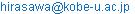(S. Hirasawa)

*Corresponding author

Shigeki Hirasawa, Tsuyoshi Kawanami, Katsuaki Shirai. Thermal Performance and Cost to Produce 90°C-Hot Water of Solar Thermal Collector and Hybrid Photovoltaic Thermal (PVT) Collector Systems. International Journal of Energy and Power Engineering. Vol. 5, No. 4, 2016, pp. 155-162. doi: 10.11648/j.ijepe.20160504.13

Received: August 8, 2016; Accepted: September 6, 2016; Published: September 19, 2016

Abstract: The effects of types of collector systems, operation-control methods, and locations in the world on thermal performance of solar thermal collector systems and cost to produce required amount of 90°C-hot water were studied through numerical simulations. Also performance of a hybrid photovoltaic thermal (PVT) collector system was examined. Calculation results showed that the costs for all solar thermal collector systems were much less than that without solar thermal collector system. Effect of the locations in the word on the cost was large. Total energy efficiency and exergy efficiency of PVT system were lower than those of the solar thermal collector system. The cost for PVT system was higher than that for the solar thermal collector system.

Keywords: Thermal Efficiency, Exergy, Cost, Solar Thermal Collector, Hybrid Photovoltaic Thermal Collector

1. Introduction

Alternative energy systems have been developed to reduce consumption of fossil energy and to decrease CO2 emissions. Solar energy is one of these clean energies. If every house used more solar energy for their heat source and electrical energy source, much more fossil energy could be saved. Three types of solar energy utilization systems were developed: a solar thermal collector system, a photovoltaic (PV) power generation system, and a hybrid photovoltaic thermal (PVT) collector system. Solar thermal collector systems, that enable hot water to reach 42°C for baths, are presently widely used in Japan. It is important to develop highly efficient solar thermal collector systems to obtain 90°C-hot water to expand the usage of solar thermal energy. Also PV systems are widely used. Recently PVT system has been studied because its total energy efficiency is higher than that of PV system.

Some works on solar thermal collector systems have been reported. Kovarik et al.  reported the optimal control of water flow through collectors. Dorato  reported the dynamic optimization techniques to design solar energy temperature control system. Hahne  reported the effect of parameters on the efficiency of flat-plate collector. Krause et al.  reported the optimization procedure to improve performance of large solar thermal system. Andersen et al. , Robles et al.  and Wrobel et al.  reported the effect of weather and location on thermal performance of solar thermal collector systems. Authors [8 - 11] studied highly efficient flat-plate evacuated solar thermal collector systems.

Also some works on PVT systems have been reported. Dubey et al.  reported the experimental results of thermal and electrical efficiencies for two types of PVT systems in Singapore. Evola et al.  reported the analytical results of exergy and thermoeconomic optimization of PVT system. Shyama et al.  reported the analytical results of two configurations of PVT system on thermal efficiency and electrical efficiency. Lammle et al.  reported the experimental results of PVT system with low-emissivity coating on the solar panel.

In this work, the effects of parameters (types of collector systems, operation-control methods, and locations in the world) on the thermal performance of the solar thermal collector systems and the cost to produce required amount of 90°C-hot water were studied through numerical simulations. Also performance of PVT system was examined and compared with those of the solar thermal collector systems.

2. Calculation Method for Solar Thermal Collector Systems and PVT Systems

Two types of solar thermal collector systems were studied: an accumulating heated water collector system, and a water-circulating collector system. Figure 1 shows the accumulating heated water collector system, which supplies new water from morning to evening and accumulates heated water in a heat storage tank. There is no water in the heat storage tank in the morning. On the other hand the water-circulating collector system circulates water between the collector plate and the heat storage tank. Some water is in the heat storage tank in the morning and the water is heated over time by circulating it.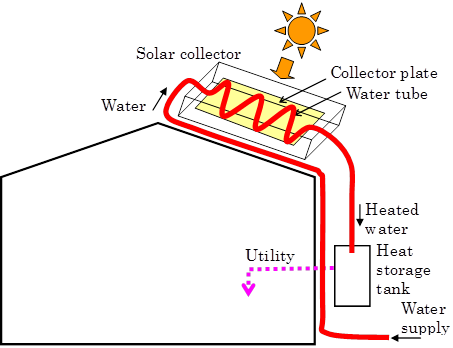Figure 1. Accumulating heated water system.

Figure 2 outlines the calculation model for a flat-plate evacuated solar thermal collector. A selective absorption film was coated on the upper side of the collector plate . The calculation conditions were as follows. Six collector plates of 1 m2 were used and the total collection area of the collector plates was 6 m2. The collector plates were aluminum with a thickness of 0.5 mm and their inclined angles were 30°. The air pressure in the collectors was vacuum pressure of 0.1 Pa and the water tube was attached to the back surface of the collector plate. There was Urethane wool below the collectors and the total length of water piping outside the collector plate was 10 m. The heat storage tank was 0.8 ´ 0.8 ´ 0.8 m, and there was Urethane wool with thickness of 70 mm outside the piping and the heat storage tank.

The temperature distribution in the collector plate is calculated with the following unsteady thermal conduction equation: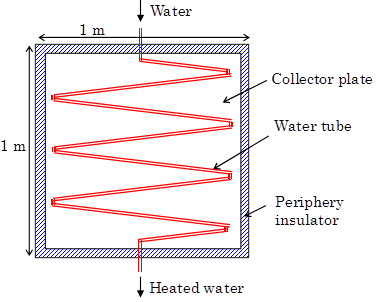(a) Top view.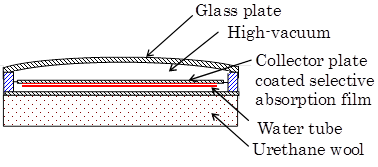(b) Vertical cross section.

Figure 2. Flat-pate evacuated solar thermal collector.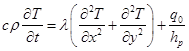(1)

where T is the temperature, t is the time, λ is the thermal conductivity, c is the specific heat, ρ is the density, hp is the thickness of the collector plate, and q0 is the heat transfer rate per unit area. The heat transfer rate per unit area q0 is calculated as:

q0 = q1 - q2 - q3 - q4 - q5 (2)

where q1 is the absorbed solar radiation, q2 is the upward radiation loss, q3 is the upward conduction loss, q4 is the backward conduction loss, and q5 is the heat transfer rate to the water. The absorbed solar radiation q1 is calculated as:

q1 = qsol τg ε1 (3)

where qsol is the solar radiation outside the collector system, τg is the transmissivity of glass plate (τg = 0.93), and ε1 is the absorptivity of the selective absorption film on the collector plate for solar radiation (ε1 = 0.95). The solar radiation qsol includes the effect of transient change of incidence angle of solar radiation beam on the fixed collector plate. The glass plate was assumed to be opaque to infrared radiation. Upward radiation loss q2 is calculated as: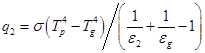(4)

where ε2 is the emissivity of the selective absorption film for infrared radiation (ε2 = 0.07), εg is the emissivity of the glass plate for infrared radiation (εg = 0.95), and σ is the Stefan-Boltzmann constant. Here, Tp and Tg are the temperatures of the collector plate and glass plate. The upward conduction loss q3 is calculated as:

q3 = (λa/ha)(Tp - Tg)  (5)

where λa is the thermal conductivity of vacuumed air (λa = 0.0037 W/m K at 0.1 Pa), and ha is the average distance between the upward surface of the collector plate and the glass plate (ha = 40 mm). The backward conduction loss through wool q4 is calculated as:

q4 = (λu/hu)(Tp - Ta)              (6)

where λu is the thermal conductivity of wool (λu = 0.024 W/m K), hu is its thickness (hu = 100 mm), and Ta is the surrounding air temperature. The forced convective heat transfer coefficient in the water tube to calculate the heat transfer rate to the water q5 was the Colburn's equation . It was assumed that water would not boil even though it temporarily reached higher than 100°C. Conduction heat losses through the Urethane wool from the piping and the heat storage tank were also calculated. The initial amount of water in the tank at 8:00 for the water-circulating type was determined so that the temperature in the tank at 17:00 reached 90°C on sunny days of each month in advance. The water flow rate in the accumulating heated water system without control was determined so that the amount of water in the tank at 17:00 would be same as that in the water-circulating system. These equations were numerically calculated using the implicit finite difference method . The calculation time step was 60 s.

Figure 3 shows the vertical cross section of PVT collector. There was a glass plate above the photovoltaic panel. Air pressure in the collectors was atmospheric pressure. There was an aluminum collector plate with water tube on the back surface of the photovoltaic panel.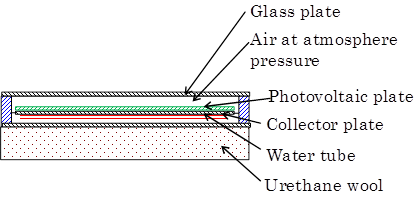Figure 3. Hybrid photovoltaic thermal (PVT) collector systems.

Transient electric power generation efficiency hel of the photovoltaic panel is calculated as follows :

ηel = ηel-room {1.0 - βT (TPV - Troom)}      (7)

where ηel-room is the efficiency of photovoltaic panel at room temperature (ηel-room = 0.182), βT is the temperature coefficient (βT = 0.0041 K-1), TPV is the photovoltaic panel temperature, and Troom is the room temperature (Troom = 298 K). The emissivity of the photovoltaic panel for infrared radiation is ε2 = 0.6 for the upward radiation loss q2 in Eq. (4), because there is no selective absorption film on the photovoltaic panel. The thermal conductivity of air at atmospheric pressure is λa = 0.023 W/m K for the upward conduction loss q3 in Eq. (5). Other calculation conditions are similar to the solar thermal collector. The heat transfer rate per unit area q0 of the collector plate is as follows:

q0 = q1 - q2 - q3 - q4 - q5 - q6          (8)

q6 = qsol τg ηel                (9)

where q6 is the electric power generation rate per unit area.

The thermal efficiency ηth of the collectors for one day is defined as the ratio between the total accumulated heat of water in the heat storage tank at 17:00 and the total solar radiation energy Qsol for one day.

ηth = Gw c (Ttank - Ta) / Qsol          (10)

where Gw is the amount of water in tank at 17:00, c is the specific heat of water, Ttank is the water temperature in the heat storage tank at 17:00, and Ta is the average surrounding air temperature. Electrical efficiency ηel for one day and total energy efficiency ηtotal are calculated as follows:

ηel = Pel / Qsol                 (11)

ηtotal = ηth + ηel                 (12)

where Pel is the total electric power for one day.

Exergy Eth of the accumulated heat of water in the heat storage tank at 17:00 is calculated as follows :

Eth = Gw c {(Ttank - Troom) - Troom ln(Ttank / Troom)}  (13)

Exergy Eel of the total electric power Pel for one day is calculated as follows :

Eel = Pel                  (14)

Exergy Esol of the total solar radiation energy Qsol for one day is calculated as follows :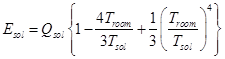(15)

where Tsol is the apparent solar temperature (Tsol = 5760 K).

Exergy efficiency ηex for one day is calculated as follows:

ηex = (Eel + Eth) / Esol              (16)

The cost to produce the required amount 90°C-hot water was calculated as following. The required amount of heated water was assumed to 331 kg with a temperature 90°C at 17:00 for every day, which was the value of the collected hot water on the sunny day on April 2 using the water-circulating system. If the collected amount or the temperature of heated water was less than that required, the collected water was added to the deficient amount and heated to the required temperature with a gas boiler. The heating cost of the gas boiler was assumed to be 5´10-6 ¥/J, the running cost of the pump for a flow rate of 1 kg/s was 27 ¥/day, and the initial cost of the collector equipment and controller were 82 ¥/day and 27 ¥/day, which are typical values in Japan.

3. Calculation Results for Solar Thermal Collector Systems

3.1. Effect of Collector System and Operation Control

Figure 4 plots the daily changes in solar radiation qsol and surrounding air temperatures Tair evaluated in this research on April 1, 2012 in the city of Osaka in Japan, which were obtained from the database of the Japan Meteorological Agency . Conditions for April 2 - 5, August 1, October 2 and January 2 were also examined and the averages for the eight typical weather conditions of four seasons were examined.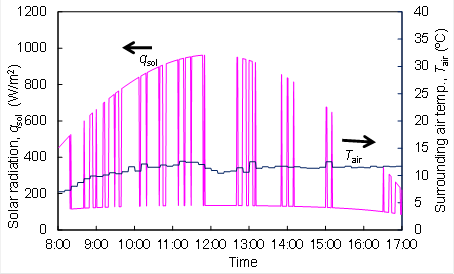Figure 4. Solar radiation and surrounding air temperature on April 1 in Osaka.

Figure 5 plots the calculated results for the outlet-temperature of the heated water from the collector plate Tout, temperature in the heat storage tank Ttank and transient thermal efficiency ηth on April 1 using the accumulating heated water system without control in the Osaka. As the transient thermal efficiency ηth changed very rapidly, average values for 10 minutes were shown in Figure 5.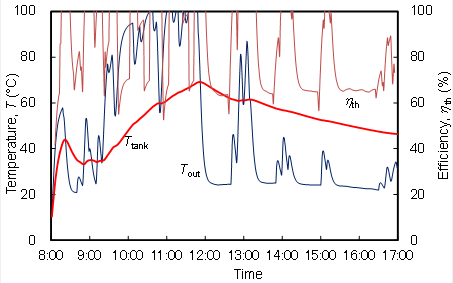Figure 5. Temperature change and thermal efficiency for accumulating heated water system on April 1.

Figure 6 plots the calculated results for the water-circulating system without control on April 1.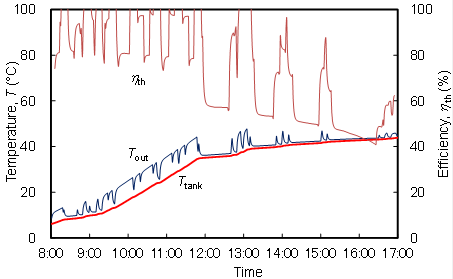Figure 6. Temperature change and thermal efficiency for water-circulating system on April 1.

The effects of operation-control on the thermal performance of collectors were calculated for the accumulating heated water system. Two operation-control methods were examined: the flow rate of the water was controlled to keep a constant outlet-temperature of the heated water, and the flow rate of the cooling water was controlled proportionally to match the solar radiation rate. Figures 7 and 8 plot the calculated results for temperature change for two operation-control methods. Temperature in the heat storage tank Ttank became high at 10:00 with operation-control, which was faster than that without control shown in Figure 5.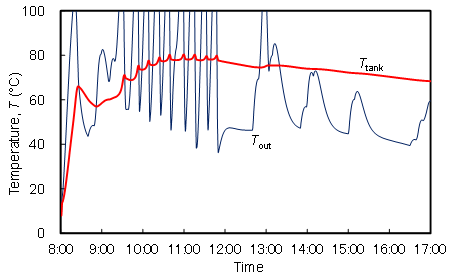Figure 7. Temperature change for control with water temperature using accumulating heated water system on April 1.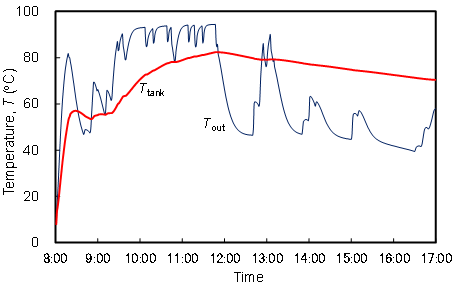Figure 8. Temperature change for control with solar radiation using accumulating heated water system on April 1.

Figures 9 and 10 plot the calculated results of the average temperature Ttank12-15 in the heat storage tank from 12:00 - 15:00, the temperature Ttank17 in the heat storage tank at 17:00, thermal efficiency hth, and cost to produce the required amount of heated water for various collector systems and operation-control methods. The values in Figures 9 and 10 are the averages for the eight typical weather conditions of four seasons in Osaka. The thermal efficiency hth and temperature Ttank17 in the heat storage tank at 17:00 were almost the same for all solar thermal collector systems. The temperature of collected water with control was slightly higher than that without control but the thermal efficiency was slightly lower. We also showed the cost to produce the required amount of heated water with a gas boiler without a solar collector system in Figure 10. The costs to produce the required amount of heated water by the all solar thermal collector systems were much less than that without solar thermal collector systems, which indicates the flat-plate evacuated solar thermal collector systems are beneficial in energy savings and cost savings.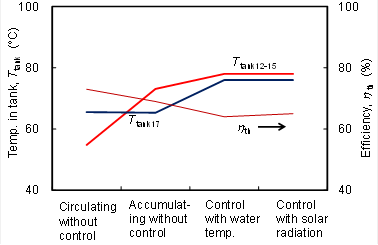Figure 9. Temperature in tank and thermal efficiency for various collector systems and operation-control methods.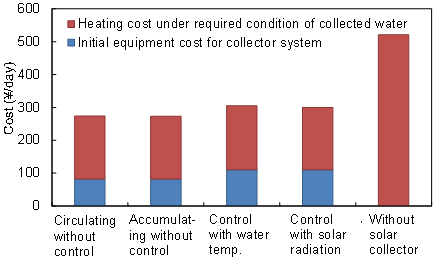Figure 10. Cost for various collector systems and operation-control methods.

3.2. Effect of Weather Conditions at Various Locations in the World

The effects of weather conditions at various locations in the world were examined. Figure 11 plots the average daily changes in solar radiation qsol and surrounding air temperature Tair in April in Berlin in Germany, Osaka in Japan, California in USA and Delhi in India, which were obtained from the database of the U.S. Department of Energy . Conditions for August and January were also examined.

Figure 12 plots the calculated results for temperature in tank Ttank17 at 17:00 for accumulating heated water system without control at various months and various locations. Figures 13 and 14 show the calculated results of the average temperature Ttank12-15 in the heat storage tank from 12:00 - 15:00, the temperature Ttank17 in the heat storage tank at 17:00, thermal efficiency ηth, and cost to produce the required amount of heated water for the accumulating heated water system without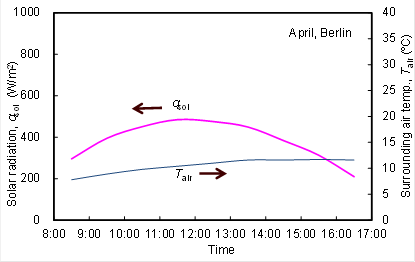(a) Berlin in Germany.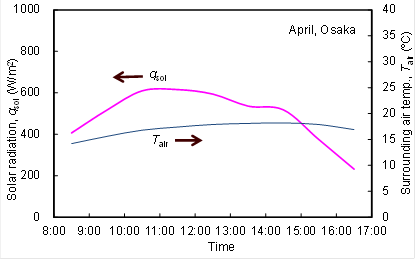(b) Osaka in Japan.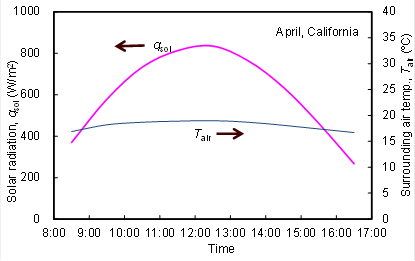(c) California in USA.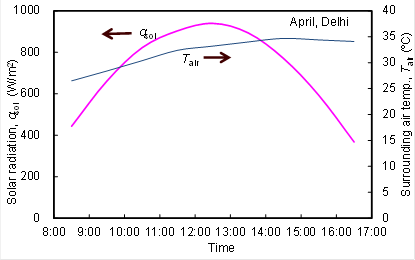(d) Delhi in India.

Figure 11. Average solar radiation and surrounding air temperature in April at various locations.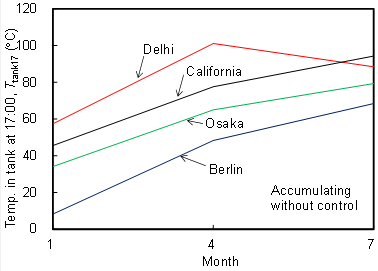Figure 12. Temperature in tank at 17:00 at various months and various locations for accumulating heated water system.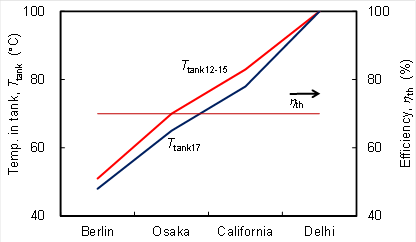Figure 13. Temperature in tank and thermal efficiency at various locations for accumulating heated water system in April.

control in April at various locations. Effect of the locations in the world on the thermal performance and cost was very large.

4. Calculation Results for Hybrid Photovoltaic Thermal (PVT) Collector System

Next, the performance of for PVT system was examined for the solar radiation condition shown in Figure 4 on April 1 in the Osaka using the accumulating heated water system without control. Figure 15 plots the calculated results for the outlet-temperature of the heated water from the collector plate Tout, temperature in the heat storage tank Ttank, transient electrical efficiency ηel, and thermal efficiency ηth.

Figures 16 - 18 plot the calculated results of the average temperature Ttank12-15 in the heat storage tank from 12:00 - 15:00, temperature Ttank17 in the heat storage tank at 17:00, electrical efficiency ηel for one day, thermal efficiency ηth, total energy efficient ηtotal, exergy efficiency ηex, and cost to produce required hot water. The values in Figures 16 - 18 are the averages for the eight typical weather conditions for the four seasons in Osaka. The results for PVT system were compared with those for the thermal collector system. Thermal efficiency ηth of PVT system was less than half of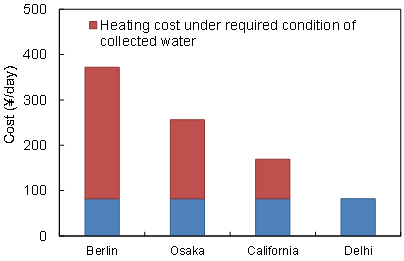Figure 14. Cost to produce required hot water at various locations for accumulating heated water system.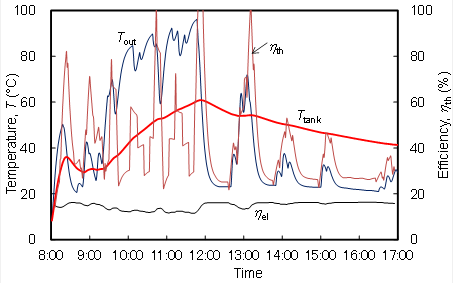Figure 15. Temperature change and thermal efficiency for PVT system on April 1.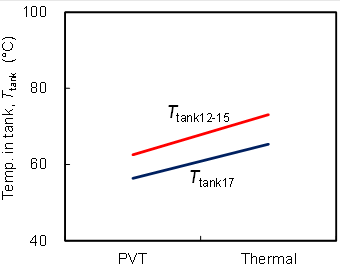Figure 16. Temperature in tank for PVT and thermal collector systems.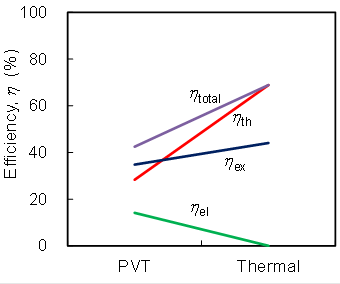Figure 17. Electrical efficiency, thermal efficiency, total energy efficiency and exergy efficiency for PVT and thermal collector systems.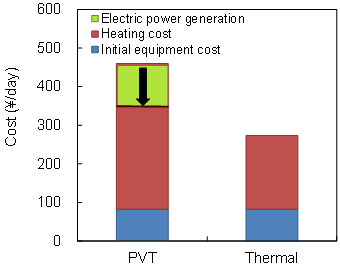Figure 18. Cost to produce required hot water for PVT and thermal collector systems.

that of the thermal collector system because the upward radiation loss q2 and the upward conduction loss q3 were large. Total energy efficiency ηtotal and exergy efficiency ηex of PVT system were lower than those of the thermal collector system. The cost of PVT system was higher than that of the thermal collector system even though income profit by selling electric power generation. Electric power of PVT system is good usability compared with thermal energy, and the total energy efficiency of PVT system is higher than that of PV system. However the efficiency and cost of PVT system are unfavorable compared with those of the solar thermal collector systems.

5. Conclusions

Thermal performance of collectors and cost to produce the required amount of 90°C-hot water with evacuated solar thermal collector systems and PVT system were studied. Following results were obtained. The total cost to produce the required amount of 90°C-hot water for all solar thermal collector systems were much less than that without them. Effect of locations in the world on the cost was very large. Thermal efficiency, total energy efficiency and exergy efficiency of PVT system were lower than those of thermal collector system. Cost of PVT system was higher than that for the thermal collector system even though income profit by selling electric power generation.

Acknowledgments

Authors thank to Mr. Yuki Matsumoto, Mr. Toshihiro Inada, and Mr. Atsushi Shirai of Kobe University for helping analysis.

References

1. M. Kovarik, P. F. Lesse, "Optimal Control of Flow in Low Temperature Solar Heat Collector," Solar Energy, vol. 18 , 1976 , pp. 431-435 .
2. P. Dorato, "Optimal Temperature Control of Solar Energy Systems, " Solar Energy, vol. 30 (2) , 1983 , pp. 147-153.
3. E. Hahne," Parameter Effects on Design and Performance of Flat Plate Solar Collectors, " Solar Energy, vol. 34 (6) , 1985 , pp. 497-503.
4. M. Krause, K. Vajen, F. Wiese, H. Ackermann, "Investigations on Optimizing Large Solar Thermal Systems," Solar Energy, vol. 73 (4) , 2002 , pp. 217-225.
5. E. Andersen, S. Furbo, "Theoretical Variations of the Thermal Performance of Different Solar Collectors and Solar Combi Systems as Function of the Varying Yearly Weather Conditions in Denmark," Solar Energy, vol. 83 (4) , 2009 , pp. 552-565.
6. A. Robles, V. Duong, A. J. Martin, J. L. Guadarrama, G. Diaz, "Aluminium Minichannel Solar Water Heater Performance under Year-Round Weather Conditions," Solar Energy, vol. 110 (12) , 2014 , pp. 356–364.
7. J. Wrobel, P. S. Walter, G. Schmitz, "Performance of a Solar Assisted Air Conditioning System at Different Locations," Solar Energy, vol. 92 (6) , 2013 , pp. 69-83.
8. S. Hirasawa, T. Kawanami, Y. Okawa, A. Shirai, "Effect of Operation-Control Methods on Collector Thermal Performance of Flat-Plate-Type Evacuated Solar Collector System to Get Hot Water near 100°C," Proc. of 22th International Symposium on Transport Phenomena, Paper-No. 11, 2011.
9. S. Hirasawa, S. Sato, T. Kawanami, "Study on Heat Transfer Characteristics of Loop Heat Pipe for Solar Collector," Transaction on Control and Mechanical Systems, vol. 2 (4), 2013 , pp. 149-154.
10. S. Hirasawa, R. Tsubota, T. Kawanami, K. Shirai, "Reduction of Heat Loss from Solar Thermal Collector by Diminishing Natural Convection with High-Porosity Porous Medium," Solar Energy, vol. 97 , 2013 , pp. 305-313.
11. S. Hirasawa, T. Kawanami, K. Shirai, "Optimal System Design and Operation-Control Method for Evacuated Solar Thermal Collector System to Obtain Hot Water near 100°C," Proc. of International Conference on Engineering and Applied Sciences Optimization, No. 3074, 2014.
12. S . Dubey, A . A. O. Tay , "Testing of Two Different Types of Photovoltaic–Thermal (PVT) Modules with Heat Flow Pattern under Tropical Climatic Conditions," Energy for Sustainable Development, vol. 17, 201 3, pp. 1 - 12 .
13. G. Evola, L. Marletta , "Exergy and Thermoeconomic Optimization of a Water-Cooled Glazed Hybrid Photovoltaic/Thermal (PVT) Collector," Solar Energy, vol. 107, 201 4, pp. 12 - 25 .
14. Shyama, G. N. Tiwari, I. M. Al-Helal , "Analytical Expression of Temperature Dependent Electrical Efficiency of N-PVT Water Collectors Connected in Series," Solar Energy, vol. 114, 201 5, pp. 61 - 76 .
15. M . Lammle, T. Kroyer, S . Fortuin, M . Wiese, M . Hermann , "Development and Modelling of Highly-Efficient PVT Collectors with Low-Emissivity Coatings," Solar Energy, vol. 130, 201 6, pp. 161 - 173 .
16. Blue Tec Gmb H & Co., Technical information, Available at: http://www.bluetec-germany.de/fileadmin/user_upload/pdf/produktinfos/60609_echn_doku_eng.pdf.
17. J. P. Holman, Heat Transfer, 9th Ed., McGraw-Hill International Book Co., Boston, 2002.
18. R. P. Collins, E. G. Miravete, "Mathematical Model of a Hybrid Solar Panel," Proc. of ASME 2014 International Mechanical Engineering Congress and Exposition, IMECE 2014-37259, 2014.
19. Japan Meteorological Agency, Weather, Climate & Earthquake Information, Available at: http://www. jma.go.jp/jma/ indexe.htm.
20. U.S. Department of Energy, Energy Plus Energy Simulation Software, Available at: http://www.eere. energy.gov/buildings/ energyplus/cfm/weather_data.cfm.

 Contents 1. 2. 3. 3.1. 3.2. 4. 5.
Article ToolsAbstractPDF(435K)